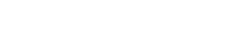# Tube Bend CalculatorCentre Line Radius   Degree of Bend           Bend allowance = Useful Information CLR = Centre Line Radius. DOB = Degree Of Bend. Length of tube in a bend = CLR X DOB X .01745 or, to put it another way: L = (R X .01745) x Z, L is the length, R is radius of the pipe bend, Z is the angle of the bend. Setback = radius X tangent ½ angle of bend. Circumference = 3.1416 X diameter.

## Related Posts### To Diff Or Not To Diff

April 8, 2020